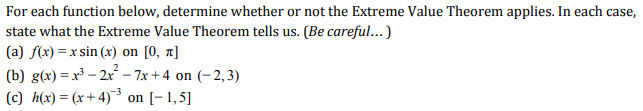# For each function below, determine whether or not the Extreme Value Theorem applies. In each case, state what the Extreme Value Theorem tells us. (Be careful...) (a) f(x)-x sin (x) on [0, π] (b) g(r)--2 -7x+4 on (-2,3) (c) h(x) = (x + 4)-, on [-1,5]

Questionhelp_outlineImage TranscriptioncloseFor each function below, determine whether or not the Extreme Value Theorem applies. In each case, state what the Extreme Value Theorem tells us. (Be careful...) (a) f(x)-x sin (x) on [0, π] (b) g(r)--2 -7x+4 on (-2,3) (c) h(x) = (x + 4)-, on [-1,5] fullscreen

### Want to see this answer and more?

Experts are waiting 24/7 to provide step-by-step solutions in as fast as 30 minutes!*

*Response times vary by subject and question complexity. Median response time is 34 minutes and may be longer for new subjects.
Tagged in
MathCalculus

### Derivative